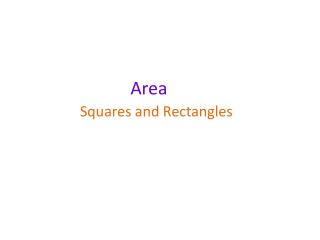DownloadDownload PresentationArea

# Area

Download Presentation## Area

- - - - - - - - - - - - - - - - - - - - - - - - - - - E N D - - - - - - - - - - - - - - - - - - - - - - - - - - -
##### Presentation Transcript

1. Area Squares and Rectangles

2. Area Rug A Rug C Rug B The area of a shape is a measure of how much surface the shape takes up. For example, which of these rugs covers a larger surface?

3. Rectangles l w The perimeter of a rectangle with length l and width w can be written as: Perimeter = 2l + 2w or Perimeter = 2(l + w) The area of a rectangle is given as: Area = lw

4. Area of a rectangle length, l Area of a rectangle = length × width width, w = lw Area is measured in square units. For example, we can use mm2, cm2, m2 or km2. The 2 tells us that there are two dimensions, length and width. We can find the area of a rectangle by multiplying the length and the width of the rectangle together.

5. Area of a rectangle What is the area of this rectangle? 4 cm 8 cm Area of a rectangle = lw = 8 cm × 4 cm = 32 cm2

6. Squares l When the length and the width of a rectangle are equal we call it a square. A square is just a special type of rectangle. The perimeter of a square with length l is given as: Perimeter = 4l The area of a square is given as: Area = l2

7. Area of shapes made from rectangles How can we find the area of this shape? We can think of this shape as being made up of two rectangles. 7 m Either like this … A 10 m … or like this. 15 m 8 m Label the rectangles A and B. B 5 m Area A = 10 × 7 = 70 m2 15 m Area B = 5 × 15 = 75 m2 Total area = 70 + 75 = 145 m2

8. Area of shapes made from rectangles How can we find the area of the shaded shape? We can think of this shape as being made up of one rectangle cut out of another rectangle. 7 cm A 3 cm Label the rectangles A and B. 8 cm B 4 cm Area A = 7 × 8 = 56 cm2 Area B = 3 × 4 = 12 cm2 Total area = 56 – 12 = 44 cm2